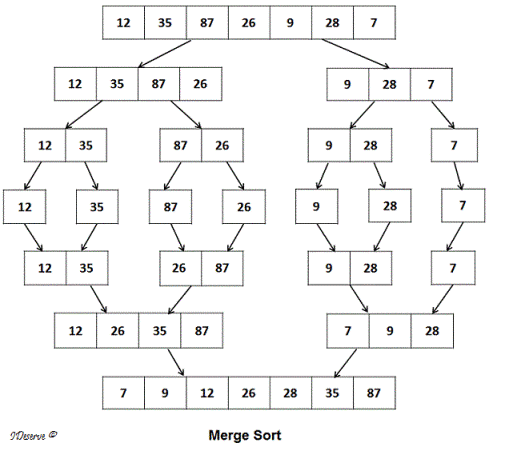# Merge Sort

Given an integer array, sort the array using Merge Sort algorithm.
Example:
Array:  12, 35, 87, 26, 9, 28, 7
Output: 7, 9, 12, 26, 28, 35, 87

Goldman Sachs

## Algorithm/Insights

Merge sort is a comparison based algorithm that uses divide and conquer approach to sort an array in O(n logn) time.
Merge sort is a stable sort i.e. it preserves the order of same array elements after sorting.

Algorithm for Merge sort is:
Step 1. Divide the array into 2 halves recursively.
Step 2. Merge the divided parts in sorted order.

Here is an example that explains the algorithm:First, the array {12, 35, 87, 26, 9, 28, 7} is divided to sub arrays {12, 35, 87, 26} and {9, 28, 7} as shown in the image above.
Then {12, 35, 87, 26} is divided into {12, 35} and {87, 26}.
Next {12, 35} is divided into {12} and {35}
At this step, no further division is possible on single element arrays {12} and {35}.
Next step is to merge these arrays in sorted order to get {12, 35}

Next {87, 26} is divided into {87} and {26}
Similar as above, we merge these single element arrays in sorted order to get {26, 87}

Now we are at the step where we have {12, 35} and {26, 87}. We merge these subarrays in sorted order to form sorted array: {12, 26, 35, 87}

Similarly, {9, 28, 7} is also first divided recursively and then merged in sorted order to get sorted array {7, 9, 28}

Finally, we merge sorted subarrays {12, 26, 35, 87} and {7, 9, 28} in sorted order to get the sorted array {7, 9, 12, 26, 28, 35, 87}.

Here is the algorithm for merging 2 sorted arrays used by merge sort algorithm given in the code snippet section:
1. Create temporary arrays temp1 and temp2.
2. Copy elements of first subarray to temp1. So, temp1 = {12, 26, 35, 87}
3. Copy elements of second subarray to temp2. So, temp2 = {7, 9, 28}
4. Now copy elements from temp1 and temp2 to the original array in sorted order:
a. Traverse arrays temp1 and temp2 together:
b. If temp1[i] <= temp2[j], then copy temp1[i] to the original array and move i to next element in temp1.
c. Else copy temp2[j] to the original array and move j to next element in temp2.
d. If end of one temporary array is reached, then copy the elements of the other temporary array to the original array in the same order.

## Code Snippet

```
package com.ideserve.questions.saurabh;

import java.util.Arrays;

/**
* <b>IDeserve <br>
* Given an array, sort the array using Merge Sort algorithm.
*
* @author Saurabh
*/
public class MergeSort {

public static void mergeSort(int[] array) {
mergeSort(array, 0, array.length-1);
}

private static void mergeSort(int[] array, int start, int end) {
if(start < end) {
int mid = (start+end)/2;
mergeSort(array, start, mid);
mergeSort(array, mid+1, end);
merge(array, start, mid, end);
}
}

private static void merge(int[] array, int start, int mid, int end) {
int n1 = mid - start + 1;
int n2 = end - mid;

int[] temp1 = new int[n1];
int[] temp2 = new int[n2];

for(int i = 0; i < n1; i++) {
temp1[i] = array[start+i];
}

for(int j = 0; j < n2; j++) {
temp2[j] = array[mid+j+1];
}

int i = 0, j = 0, k = start;
while(i < n1 && j < n2) {
if(temp1[i] <= temp2[j]) {
array[k] = temp1[i];
i++;
} else {
array[k] = temp2[j];
j++;
}
k++;
}

while(i < n1) {
array[k] = temp1[i];
i++;
k++;
}
while(j < n2) {
array[k] = temp2[j];
j++;
k++;
}
}

public static void main(String[] args) {
int[] array = {12, 35, 87, 26, 9, 28, 7};
mergeSort(array);
System.out.println(Arrays.toString(array));
}
}
```

## Order of the Algorithm

Time Complexity is O(n log n) in worst, best and average cases.
Space Complexity is O(n)